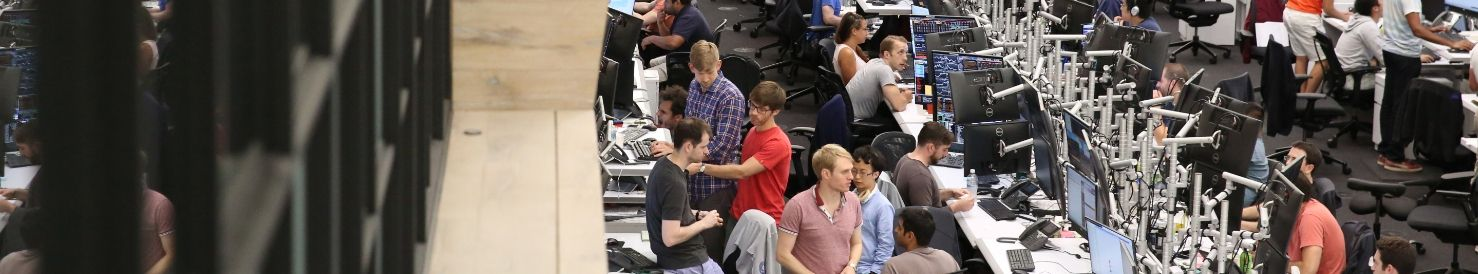Jane Street

## Sollicitatievraag

Sollicitatiegesprek voor de functie Quantitative Researcher

-

# Game: I throw 1 die 4 times, trying to reach at least one 6, you throw 2 dice 24 times and try to reach at least one double 6 (6,6). Who has greater chance of winning

## Antwoorden op sollicitatievragen

10 antwoorden

9

To estimate, compare (5/6)^4 and (35/36)^24 this is 5/6 and (35/36)^6 this is 30/36 and (35/36)^6 notice that 30/36 is missing six 1/6th from 1 (36/36) and taking powers of (35/36)^6 will reduce the number by nearly 1/36th each time, but less than than, so that (35/36)^6 is greater than 1-6/36=30/36. Therefore the probability of not getting any double six is greater than probability of not getting any 6, and you should choose to roll one die. To understand the reasoning, think about taking powers of 0.90, 0.90^1 = 1-1x0.10 0.90^2 > 1-2x0.10 0.90^3 > 1-3x0.10 and so on

Deen op

8

It all comes to which is greater: 1-(5/6)^4 or 1-(35/36)^24. They will expect you to calculate this (which is greater, not actual numbers) without a calculator.

Anoniem op

1

First comment is correct, second comment is wrong since it asks for at least one 6 or at least one (6,6). This also includes the outcomes 2 or more sixes or double (6,6). Hence the easiest way of calculating this is by calculating the complementary probabilities P(no six) and P(no double six), respectively, to get P(at least one six) = 1 - P(no six) and P(at least one double 6) = 1 - P(no double six), which gives the result in the first comment.

Anoniem op

0

I agree with the Deen's answer. Just to add up, basically if 1/36 is constantly decreased six times, it is equal to decreasing 1/6 one time. However, each time you decrease 1/36, the total size shrinks, so the next 1/36th decrease is smaller than 1/6. Therefore, 35/36^6 is greater than 5/6.

Student op

0

I agree with the Deen's answer. Just to add up, basically if 1/36 is constantly decreased six times, it is equal to decreasing 1/6 one time. However, each time you decrease 1/36, the total size shrinks, so the next 1/36th decrease is smaller than 1/36. Therefore, 35/36^6 is greater than 5/6.

Student op

0

Bernoulli's inequality: (1-p)^n > 1-np (35/36)^24 = ((1-1/36)^6)^4 > (1-6/36)^4 = (5/6)^4 so 1-(5/6)^4 > 1-(35/36)^4, i.e., choose the first option

blah op

0

@Try again, 1 - (35/36)^24 = .4914 So you get the opposite answer... "1-(5/6)^4 or 1-(35/36)^24. They will expect you to calculate this (which is greater, not actual numbers) without a calculator." Is there a standard way of estimating this?

bob op

2

First answer is correct. Also comparing the too probability is easy. You just need to compare (35/36)^4 and (5/6)^4. Write 35/36 = (7/6)*(5/6) > (5/6) as 7/6 > 1 So, 1-(35/36)^24 < 1-(5/6)^4 Hence I will prefer atleast 6 option in 4 rolls.

zooboo op

0

The first comment would be correct if you needed to get a string of 6's, probability from 4 rolls of one 6 is 4/6 (4 * 1/6), probability of 6 and a 6 is 1/36, rolled 24 times = 24/36, so they are both the same odds.

Anoniem op

1

(7/6) (5/6) > (5/6) but you have to compare [(7/6) (5/6)] ^ 24 vs [5/6] ^ 4 which you might be able to guess is smaller than (5/6) ^ 4 Just for the record 1 - (5/6)^4 = .5177 1 - (35/36)^24 = .5192 so you should take the option of rolling double 6 in 24 rolls if you feel like winning

Try again. op

## Voeg antwoorden of opmerkingen toe

Meld u aan of registreer u om hier een opmerking over te maken.

## Carrières bij Jane StreetA closer look into the roles of trading and technology offers a sense of life at Jane Street. Work at Jane Street is exciting and...Meer

• Careers
• Benefits
• Around the Office
Hier krijgt de werkgever de kans om te vertellen waarom u bij zijn of haar bedrijf moet komen werken. De informatie wordt gegeven vanuit werkgeversperspectief.